Home » Solutions for Skill test: Data Science in Python

# Solutions for Skill test: Data Science in Python

## Introduction

Python is gaining ground very quickly among the data science community. We are increasingly moving to an ecosystem, where data scientists are comfortable with multiple tools and use the right tool depending on the situation and the stack.

Python offers ease of learning, large ecosystem (web development / automation etc.), an awesome community and of course multiple computing libraries. It is not only becoming the preferred tool for newbies wanting to learn data science, but also among the professional data scientists. Python offers the best eco-system, if you are looking to work on / learning deep learning.

With this in mind, it was only a matter of time that we came out with a skill test for Python. So, we conducted our first Python Skilltest on 25th September and guess what the winner came out flying, karim.lulu scoring 44 out of 45 questions!

If you use Python as your preferred tool for data science or are learning it, here is a chance to check your skills (in case you missed it). For those who took the test live, read on the to find the right questions.

## The Skill test

We got 1337 registrations for the Python skill test and more than 250 people actually made a submission.

Topics covered:

• Importing files & data Data exploration and visualization using Pandas and Matplotlib
• Basic data manipulation & data cleaning using Python
• pandas Regular Expressions

Versions of required libraries :

• Pandas – 0.18.1
• Numpy – 1.11.1
• Matplotilb – 1.5.1
• re – 2.2.1

### Overall Performance

We had 45 questions in the skill test. The winner got 44 answers right! Here is the distribution of the scores: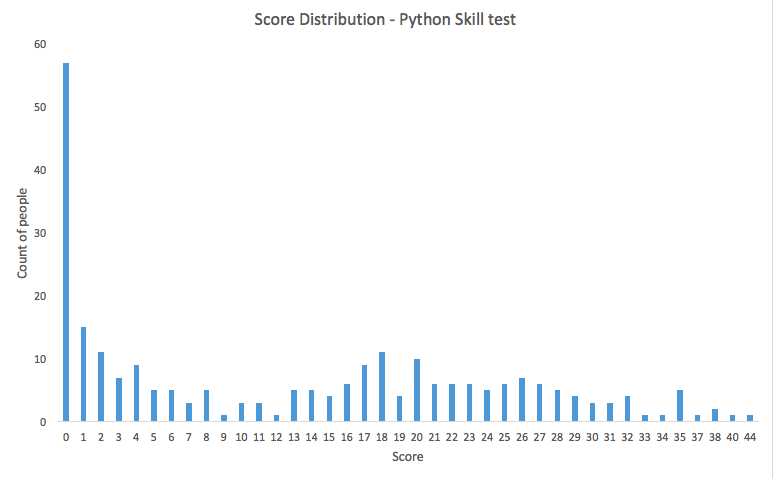Interesting distribution! Looks like our choice of questions intimidated a lot of people with a lot of them scoring 0. Overall, here is a brief summary of the performance:

• mean = 12.8095
• Median = 13
• Mode = 0

So, here are the questions along with there answers as were used in Python skill test:

## Skill test Questions and Answers

Above dataset has mix of categorical and continuous features. Every data scientist must know that handling categorical values is different from numerical values.

So how would you calculate the number of columns having categorical values?

`A - (train.dtype == 'object').sum()`
`B - (train.dtypes == object).sum()`
`C - (train.dtypes == object).count()`

D – None of these

Solution: B

Categorical variables are denoted with datatype as “object”.

Now that you have found that there are some categorical columns present in the dataset. Each categorical column may contain more than two distinct values. For example, “Married” has two values, “Yes” and “No”.

How will you find all the distinct values present in the column “Education”?

`A - train.Education.individuals()`
`B - train.Education.distinct()`
`C - train.Education.unique()`

D – None of these

Solution: C

To find all the distinct values of a particular column, the function “unique” can be used.

Further, you observe that the column “LoanAmount” has some missing values.

How can you find the number of missing values in the column “LoanAmount”?

`A - train.count().maximum() - train.LoanAmount.count()`
`B - (train.LoanAmount == NaN).sum()`
`C - (train.isnull().sum()).LoanAmount`

D – All of these

Solution: C

The function “isnull()” gives us individual boolean values of the missing values, i.e. is the value is missing or not. In python 2.7, boolean values are represented as 1 and 0 for True and False respectively. So taking their sum gives us the answer.

Next, you also see that “Credit_History” has a few missing values. You want to first analyze people who have a “Credit_History”.

You need to create a new DataFrame named “new_dataframe”, which contains rows which have a non-missing value for variable”Credit_History” in our DataFrame “train”. Which of the following commands would do this?

`A - new_dataframe = train[~train.Credit_History.isnull()]`
`B - new_dataframe = train[train.Credit_History.isna()]`
`C - new_dataframe = train[train.Credit_History.is_na()]`

D – None of these

Solution: A

The “~” operator works as a negation operator to boolean values. So in simple terms, option A is correct.

In the dataset above, you can see row with Loan_id = LP001005 has very little information (i.e. most of the variables are missing). It is recommended to filter out these rows as they could create problems / noise in your model.

If a row contains more than 5 missing values, you decide to drop them and store remaining data in DataFrame “temp”. Which of the following commands will achieve that?

`A - temp = train.dropna(axis=0, how='any', thresh=5)`
`B - temp = train.dropna(axis=0, how='all', thresh=5)`
`C - temp = train.dropna(axis=0, how='any', thresh=train.shape - 5)`

D – None of these

Solution: C

In the “thresh” argument of “dropna” function, you have to specify the threshold after which to drop Nan values, i.e. if you want to drop rows with more than 5 missing values, you would have to subtract it with total number of columns.

Now, it is time to slice and dice data. The first logical step is to make data ready for your machine learning algorithm. In the dataset, you notice that number of rows having “Property_Area” equal to “Semiurban” is very low. After thinking and talking to your business stakeholders, you decide to combine “Semiurban” and “Urban” in a new category “City” . You also decide to rename “Rural” to “Village”

Which of the following commands will make these changes in the column ‘Property_Area’ ?

`A - >>> turn_dict = ['Urban': 'City', 'Semiurban': 'City', 'Rural': 'Village']`
`  >>> train.loc[:, 'Property_Area'] = train.Property_Area.replace(turn_dict)`
`B - >>> turn_dict = {'Urban': 'City', 'Semiurban': 'City', 'Rural': 'Village'}`
`    >>> train.loc[:, 'Property_Area'] = train.Property_Area.replace(turn_dict)`
`C - >>> turn_dict = {'Urban, Semiurban': 'City', 'Rural': 'Village'}`
`    >>> train.iloc[:, 'Property_Area'] = train.Property_Area.update(turn_dict)`

D – None of these

Solution: B

To solve, first you create a dictionary with the specified conditions, than feed it in the “replace function”

While you were progressing in direction of building your first machine learning model, you notice something interesting. On a quick overview of the first few rows, you see that percentage of people who are “Male” and are married (Married = “Yes”) seems high.

To check this hypothesis, how will you find the percentage of married males in the data?

`A - (train.loc[(train.Gender == 'male') && (train.Married == 'yes')].shape / float(train.shape))*100`
`B - (train.loc[(train.Gender == 'Male') & (train.Married == 'Yes')].shape / float(train.shape))*100`
`C - (train.loc[(train.Gender == 'male') and (train.Married == 'yes')].shape / float(train.shape))*100`

D – None of these

Solution: D

Always remember, to take multiple boolean indexing, specify with “&” operator. Also “shape” returns the total number of columns. And don’t forget the case, as python is case sensitive!

Take a brief look at train and test datasets mentioned above. You might have noticed that the columns in these datasets do not match, i.e. some columns in train are not present in test and vice versa.

How to find which cols are present in test but not in train? Assume data has already been read in DataFrames “train” & “test” respectively.

`A - set(test.columns).difference(set(train.columns))`
`B - set(test.columns.tolist()) - set(train.columns.tolist())`
`C - set(train.columns.tolist()).difference(set(test.columns.tolist()))`

D – Both A and B

Solution: D

This is a classic example of set theory.

Q 9)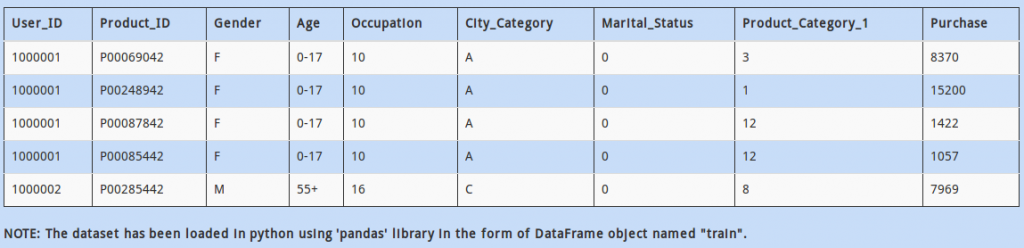As you might be aware, most of the machine learning libraries in Python and their corresponding algorithms require data to be in numeric array format.

Hence, we need to convert categorical “Gender” values to numerical values (i.e. change M to 1 and F to 0). Which of the commands would do that?

`A - train.ix[:, 'Gender'] = train.Gender.applymap({'M':1,'F':0}).astype(int)`
`B - train.ix[:, 'Gender'] = train.Gender.map({'M':1,'F':0}).astype(int)`
`C - train.ix[:, 'Gender'] = train.Gender.apply({'M':1,'F':0}).astype(int)`

D – None of these

Solution: B

(diff map, apply)

In the datasets above, “Product_ID” column contains a unique identification of the products being sold. There might be a situation when there are a few products present in the test data but not id train data. This could be troublesome for your model, as it has no “historical” knowledge for the new product.

How would you check if all values of “Product_ID” in test DataFrame are available in train DataFrame dataset?

`A - train.Product_ID.unique().contains(test.Product_ID.unique())`
`B - set(test.Product_ID.unique()).issubset(set(train.Product_ID.unique()))`
`C - train.Product_ID.unique() = test.Product_ID.unique()`

D – None of these

Solution: B

If you look at the data above, “Age” is currently a categorical variable. Converting it to a numerical field might help us extract more meaningful insight.

You decide to replace the Categorical column ‘Age’ by a numeric column by replacing the range with its average (Example: 0-17 and 17-25 should be replaced by their averages 8.5 and 21 respectively)

`A - train['Age'] = train.Age.apply(lambda x: (np.array(x.split('-'), dtype=int).sum()) / x.shape)`
`B - train['Age'] = train.Age.apply(lambda x: np.array(x.split('-'), dtype=int).mean())`

C – Both of these

D – None of these

Solution: B

A somewhat hacky approach, but it works. First you separate the string on “-” and then find its mean. (If you are wondering why option A doesn’t work, check it out! )

The other scenario in which numerical value could be “hiding in plain sight” is when it is plagued with characters. We would have to clean these values before moving on to model building.

For example, in “Ticket”, the values are represented as one or two blocks separated with spaces. Each block has numerical values in it, but only the first block has characters combined with numbers. (eg. “ABC0 3000”).

Which of the following code return only the last block of numeric values? (You can assume that numeric values are always present in the last block of this column)

`A - train.Ticket.str.split(' ').str`
`B - train.Ticket.str.split(' ').str[-1]`
`C - train.Ticket.str.split(' ')`

D – None of these

Solution: B

To index the last term of a python list, you can use “-1”

As you might have noticed (or if you haven’t, do it now!), the above dataset is the famous Titanic dataset. (PS: its a bit unclean than usual, but you get the gist, right? )

Coming back to the point, the data has missing values present in it. It is time to tackle them! The simplest way is to fill them with “known” values.

You decide to fill missing “Age” values by mean of all other passengers of the same gender. Which of the following code will fill missing values for all passengers by the above logic?

`A - train = train.groupby('Sex').transform(lambda x: x.fillna(x.sum()))`
`B - train['Age'] = train.groupby('Sex').transform(lambda x: x.fillna(x.mean())).Age`
`C - train['Age'] = train.groupby('Sex').replace(lambda x: x.fillna(x.mean())).Age`

D – None of these

Solution: B

To solve, group the data on “Sex”, and then fill all the missing values with the appropriate mean. Remember that python lambda is a very useful construct. Do try to inculcate the habit of using it.

Let’s get to know the data a bit more.

We want to know how location affects the survival of people. My hypothesis is that people from location “S” (S=SouthHampton), particularly females, are more likely to survive because they had better “survival instincts”.

The question is, how many females embarked from location ‘S’?

`A - train.loc[(train.Embarked == 'S') and (train.Sex == 'female')].shape`
`B - train.loc[(train.Embarked == 'S') & (train.Sex == 'female')].shape`
`C - train.loc[(train.Embarked == 'S') && (train.Sex == 'female')].shape`

D – None of these

Solution: B

Look at the column “Name” – there is an important thing to notice. Looks like, every name has a title contained in it. For example, the name “Braund, Mr. Owen Harris” has “Mr.” in it.

Which piece of code would help us calculate how many values in column “Name” have “Mr.” contained in them?

`A - (train.Name.str.find('Mr.')==False).sum()`
`B - (train.Name.str.find('Mr.')>0).sum()`
`C - (train.Name.str.find('Mr.')=0).sum()`

D – None of these

Solution: B

As highlighted previously, boolean value “True”  is represented by 1. So option B would be the appropriate answer.

You can see that column “Cabin” has 3 missing values out 5 sample records.

If a particular column has a high percentage of missing values, we may want to drop the column entirely. However, this might also lead to loss of information.

Another method to deal with this type of variable, without losing all information, is to create a new column with flag of missing value as 1 otherwise 0.

Which of the following code will create a new column “Missing_Cabin” and put the right values in it (i.e. if “cabin_missing” then 1 else 0)?

`A - train['Missing_Cabin'] = train.Cabin.apply(lambda x: x == '')`
`B - train['Missing_Cabin'] = train.Cabin.isnull() == False`
`C - train['Missing_Cabin'] = train.Cabin.isnull().astype(int)`

D – None of these

Solution: C

To convert boolean values to integer, you can use “astype(int)”

Let us take a look at another dataset. The data represents sales of an outlet along with product attributes.

The problem is, the dataset does not contain headers. Inspite of this, you know what are the appropriate column names. How would you read the the dataframe by specifying the column names?

`A - pd.read_csv("train.csv", header=None, columns=['Item_Identifier', 'Item_Weight', 'Item_Fat_Content', 'Item_Visibility' ])`
`B - pd.read_csv("train.csv", header=None, usecols=['Item_Identifier', 'Item_Weight', 'Item_Fat_Content', 'Item_Visibility'])`
`C - pd.read_csv("train.csv", header=None, names=['Item_Identifier' ,'Item_Weight' ,'Item_Fat_Content', 'Item_Visibility'])`

D – None of these

Solution: C

To explicitly specify column names in pandas, you can use “names” argument

Sometimes while reading the data in pandas, the datatypes of columns are not parsed correctly. To deal with this problem, you can either explicitly specify datatypes while reading the data, or change the datatypes in the dataframe itself.

Which of the following code will change the datatype of “Item_Fat_Content” column from “object” to “category”?

`A - train['Item_Fat_Content'] = train['Item_Fat_Content'].asdtype('categorical')`
`B - train['Item_Fat_Content'] = train['Item_Fat_Content'].astype('category')`
`C - train['Item_Fat_Content'] = train['Item_Fat_Content'].asdtype('category')`

D – None of these

Solution: B

“category” datatype is a new feature added to pandas.

In above data, notice that the “Item_Identifier” column has some relation with the column “Item_Type”. As the first letter of “Item_Identifier” changes, the “Item_Type” changes too. For example, notice that if the value in “Item_Identifier” starts with “F”, then all the corresponding values in “Item_Type” are eatables, whereas those with “D” are drinks.

To check this hypothesis, find all values in “Item_Identifier” that starts with “F”.

`A - train.Item_Identifier.str.starts_with('F')`
`B - train.Item_Identifier.str.startswith('F')`
`C - train.Item_Identifier.str.is_start('F')`

D – None of these

Solution: B

Use “str” function in pandas to access string functions.

Q 20)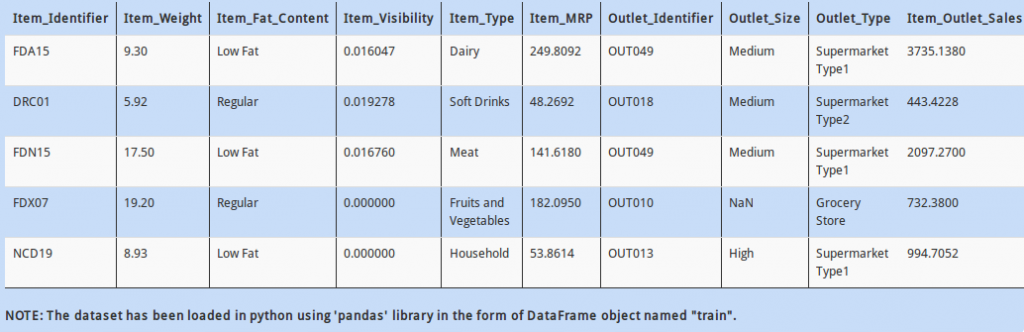Just to give your mind some rest, let us do a simple thing; convert the float values in column “Item_MRP” to integer values
`A - train['Item_MRP'] = train.Item_MRP.astype(real)`
`B - train['Item_MRP'] = train.Item_MRP.astype(int)`
`C - train['Item_MRP'] = train.Item_MRP.astype(float)`

D – None of these

Solution: B

I have another hypothesis that, if an item is more visible to new customers in a supermarket, then its more likely to be sold.

So, find correlation between “Item_Outlet_Sales” and “Item_Visibility” (use correlation method ‘pearson’)

`A - train.Item_Visibility.corr(train.Item_Outlet_Sales, method='pearson')`
`B - train.Item_Visibility.corr(train.Item_Outlet_Sales)`
`C - train.Item_Visibility.corrwith(train.Item_Outlet_Sales, method='pearson')`

D – Both A and B

Solution: D

The default argument for “method” in “corr” function is “pearson”.

Q 22)We want to check the distribution of the column ‘Hours.Per.Week’ with respect to ‘Marital.Status’ and ‘Occupation’ of the people. One thing we could do is to create a pivot table of ‘Marital.Status’ vs ‘Occupation’ and put the values.

Create the pivot table as mentioned above, with the aggregating function as “sum”

`A - train.pivot(index='Marital.Status', columns='Occupation', values='Hours.Per.Week', aggfunc='sum')`
`B - train.pivot_table(index='Marital.Status', columns='Occupation', values='Hours.Per.Week', aggfunc='sum')`
`C - train.pivot_table(index='Marital.Status', columns='Hours.Per.Week', values='Occupation', aggfunc='sum')`

D – None of these

Solution: B

(pivot_table vs pivot)

Q 23)As you can see above, the first two rows are not part of the dataset. We want to start reading from the third row of the dataset.
How would we do this using pandas in python?
`A - train = pd.read_csv('train.csv', startrow=2)`
`B - train = pd.csvReader('train.csv', startrow=2)`
`C - train = pd.read_csv('train.csv', skiprows=2)`

D – None of these

Solution: C

Q 24)Suppose the dataset is too big to be handled by your local machine, but you still want to load it into the memory. What you could do is, read only a specific number of rows, which could be easily read into the memory.

Which of the command would read only the top 500 rows?

`A - train = pd.read_csv('train.csv', nrows=500)`
`B - train = pd.read_csv('train.csv', numrows=500)`
`C - train = pd.read_csv('train.csv', rows=500)`

D – None of these

Solution: A

Q 25)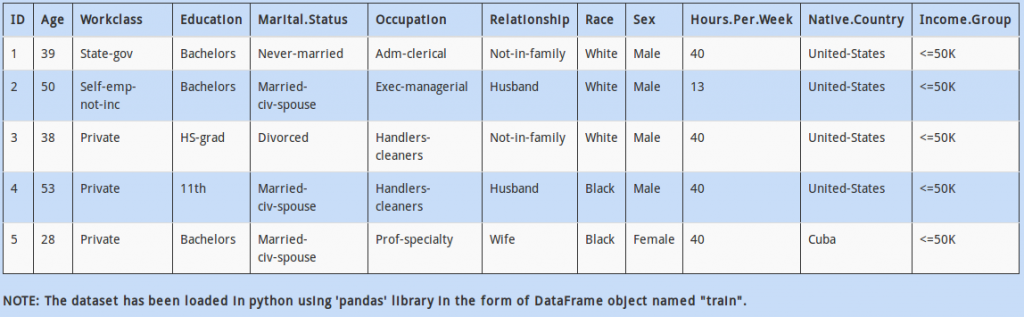Suppose we want to find how does is the column “Relationship” is distributed in the dataset. To do this, we can find the percentage distribution of population with respect to Relationship.
So, to do this find the count of corresponding Relationship status among all individuals, and then divide it by the total data points to get the percentage and map it to the original column.
`A - train['Relationship_Percentage'] = train.Relationship.map(train.Relationship.value_count/train.shape)`
`B - train['Relationship_Percentage'] = train.Relationship.map(train.Relationship.value_counts()/train.shape)`
`C - train['Relationship_Percentage'] = train.Relationship.map(train.Relationship.value_counts/train.shape)`

D – None of these

Solution: B

Q 26)Above dataframe has “Date_time_of_event” column and it is currently read as an object. This will restrict us to perform any date time operation on it.

Which command will help to convert the column “Date_time_of_event” to data type “datetime”?

`A - train['Date_time_of_event'] = pd.to_datetime(train.Date_time_of_event, date_format="%d-%m-%Y")`
`B - train['Date_time_of_event'] = pd.to_datetime(train.Date_time_of_event, format="%d-%m-%Y %H:%M")`
`C - train['Date_time_of_event'] = pd.to_datetime(train.Date_time_of_event, date_format="%d-%m-%Y %h:%m")`

D – None of these

Solution: B

Q 27)As shown above, we want to create a new column “Date” from the given “Date_time_of_event” column.

How would you you extract only the dates from the given “Date_time_of_event” column?

`A - train.Date_time_of_event.dt.days`
`B - train.Date_time_of_event.dt.day`
`C - train.Date_time_of_event.dt.Day`

D – None of these

Solution: B

Q 28)There is another thing we could do with the datetime column. We can find the name of weekday the date belongs to. How can we do this through code?
`A - train.Date_time_of_event.dt.weekdayname`
`B - train.Date_time_of_event.dt.weekday_name`
`C - train.Date_time_of_event.dt.Weekday_name`

D – None of these

Solution: B

Q 29)Sometimes the datetime column could be arranged in unix format. To extract useful information from the datetime column, you would have to convert it into usable format.

How would you do it?

`A - pd.to_datetime(train['TIMESTAMP'],unit='s')`
`B - pd.to_datetime(train['TIMESTAMP'],unit='second')`
`C - pd.to_datetime(train['TIMESTAMP'],unit='unix')`

D – None of these

Solution: A

Q 30)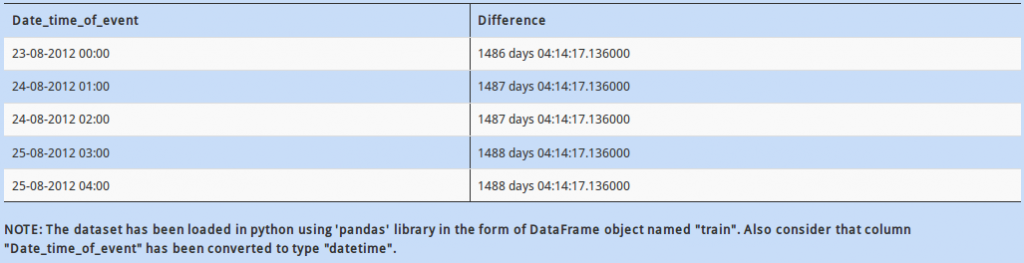Find the difference between current time and column ‘Date_time_of_event’.

`A - pd.datetime.now - train.Date_time_of_event.dt`
`B - pd.datetime.now() - train.Date_time_of_event.dt`
`C - pd.datetime.now() - train.Date_time_of_event`

D – None of these

Solution: C

Q 31)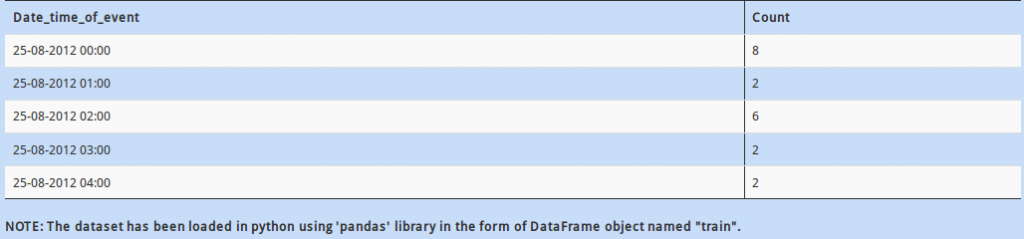Consider that you have to replace the “Date_time_of_event” column with the first day of the month.

How would you do this in python?

`A - train['Date_time_of_event'] = train.Date_time_of_event.apply(lambda x: x.replace(day=1))`
```B - >>> train['month'] = train.Date_time_of_event.dt.month; train['year'] = train.Date_time_of_event.dt.year >>> train['day'] = 1 >>> train['Date_time_of_event'] = train.apply(lambda x:pd.datetime.strptime("{0} {1} {2}".format(x['year'],x['month'], x['day']), "%Y %m %d"),axis=1)```
C – Both A and B

D – None of these

Solution: C

Q 32)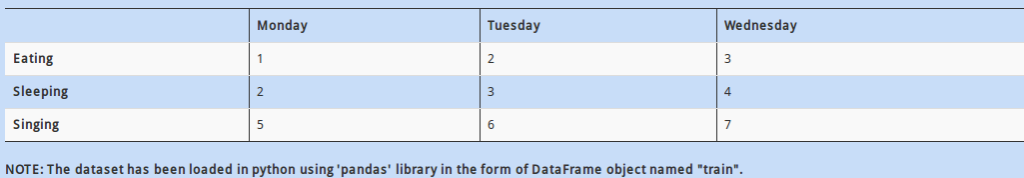The dataset above provides every day expenses on different necessities (days will be arranged in columns and expenses on necessities is in rows).

Provide python code to compute cummulative cost for each day.

`A - a.sumcum(axis=0)`
`B - a.cumsum(axis=1)`
`C - a.sumcum(axis=1)`

`D - a.cumsum(axis=0)`
Solution: B

Q 33)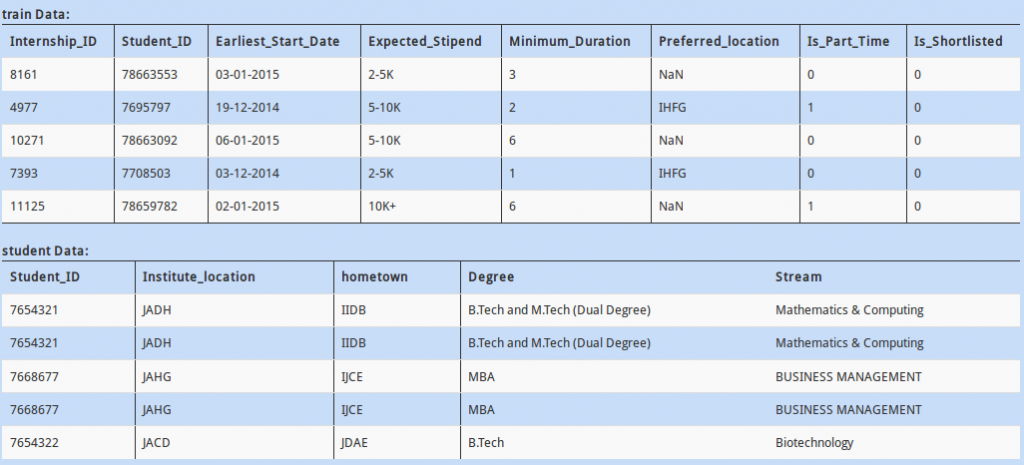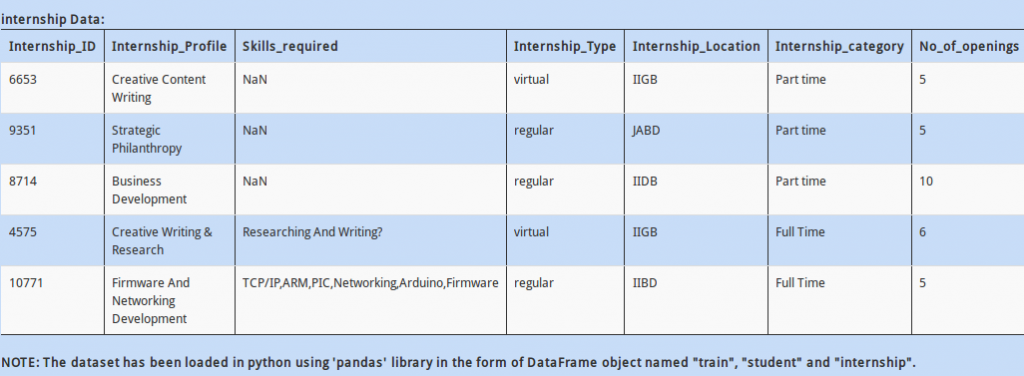For three data sets given train,student and internship we need to merge these data sets in such a way that for train data every row must have the student details from student data and intern details from intern data. (Consider only the rows which are similar in both the respective datasets)

Fill the blanks in the code:

train=pd.merge(train,internship,on=’_____’,how=’____’)
train=pd.merge(train,student,on=’_____’,how=’____’)

`A - Student_ID, outer, Internship_ID, inner`
`B - Internship_ID, right, Student_ID, inner`
`C - Internship_ID, inner, Student_ID, under`

`D - Internship_ID, inner, Student_ID, inner`
Solution: D

Q 34)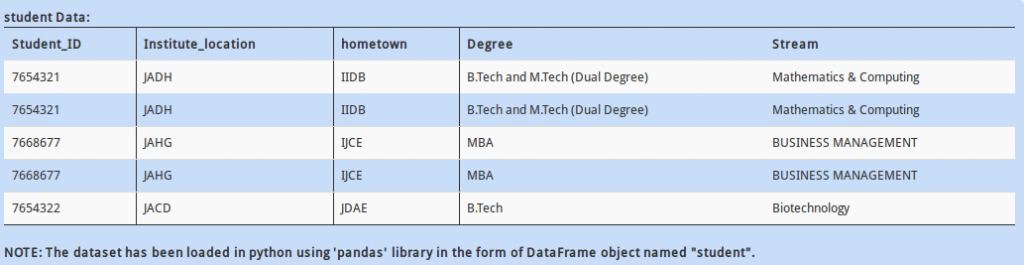In the data above, you might have noticed presence of duplicate rows. This might create problem during joins. To avoid this problem we need to remove the duplicates by keeping the first occurance only.

Fill the blanks appropriately :

student.______(subset=[‘Student_ID’],keep=_____,inplace=____)

`A - drop_same, first, True`
`B - drop_duplicates, first, False`
`C - drop_same, last, True`

`D - drop_duplicates, first, True`
Solution: D

Q 35)
Which of the following will be able to extract an e-mail address from string of words?
`A - match=re.search(r"\[email protected]\w+",string)`
`B - match=re.findall(r"[\w._][email protected][\w.]+",string)`
`C - match=re.purge(r"[\w._][email protected][\w.__]+",string)`

`D - match=re.compile(r"[\w._]@[\w.]",string)`
Solution: B

Q 36)In the data above, you want to drop the row “sleep”, how would you do it?
`A - train.drop("sleep", axis=1)`
`B - train.dropna("sleep", axis=1)`
`C - train.drop("sleep", axis=0)`D –  None of theseSolution: C

Q 37)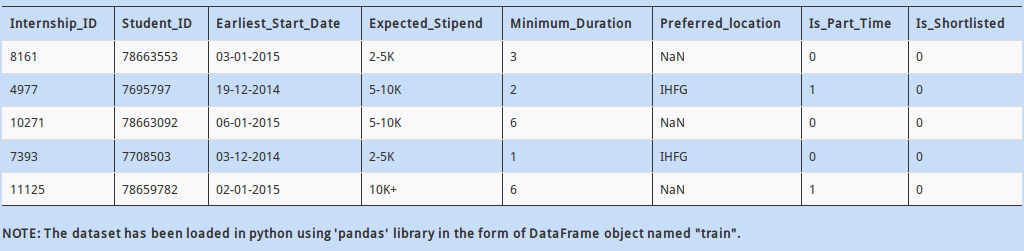Complete the code for removing the “Minimum_Duration” and “Preferred_location” variables from the “train” data set.

`train=train.drop(['Preferred_location','Minimum_Duration'],___________)`

`A - axis=0`
`B - axis=1`
`C - inplace=True`

`D - inplace=False`
Solution: B

You specify, “axis=1” when you want to access columns. On the other hand, you specify “axis=0” when accessing rows.

Q 38)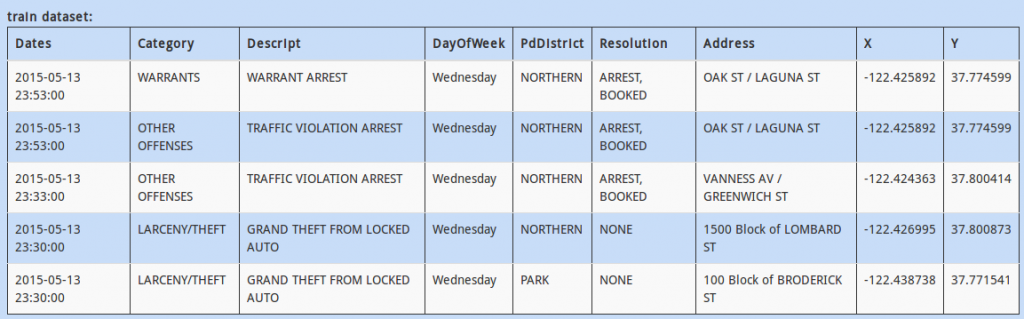For the train data set which for the different types of crimes in San Fransisco, a plot is made depicting the total number of different types of crimes with respect to “Category” column. Which of the following code will correctly give the above desired plot?
`A - train.Category.plot(kind='bar')`
`B - train.Category.hist()`
`C - train.Category.value_counts().plot(kind='bar')`D – None of theseSolution: C

Q 39)
Which of the following code will plot a stacked bar plot for relation between “Credit_History” and “Loan_Status” in the above given data set?
`A - train.unstack().plot(kind='bar',stacked=True, color=['red','blue'], grid=False)`
`B - train.restack().plot(kind='bar',stacked=True, color=['red','blue'], grid=False)`
`C - train.restack().plot(kind='bar',stacked=False, color=['red','blue'], grid=False)`

D – none of the above

Solution: A

Q 40)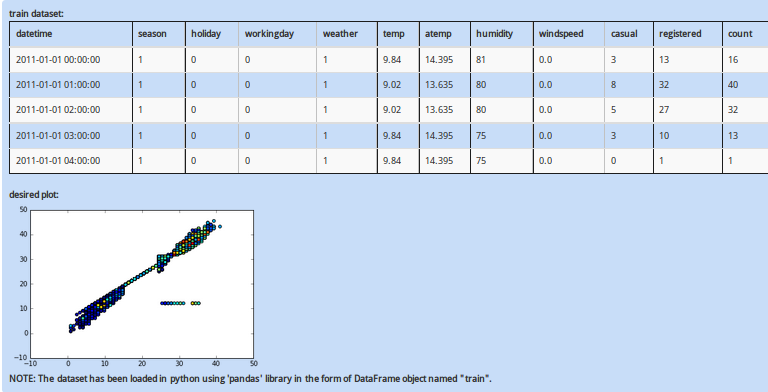A plot between “temp” and “atemp” was generated using the following code :

`plt.scatter(train.temp,train.atemp,alpha=1,c='b',s=20)`

How can we modify the code to generate a plot which will show ‘Count’ with color intensity like the graph shown above?

`A - plt.scatter(train.temp,train.atemp,alpha=1,c=train.Count.value_counts,s=20)`
`B - plt.scatter(train.temp,train.atemp,alpha=1,c=train.Count,s=20)`
`C - plt.scatter(train.temp,train.atemp,alpha=1,s=20,color=train.Count)`

`D - plt.scatter(train.temp,train.atemp,alpha=1,s=20,c=w) `

Solution: B

Q 41)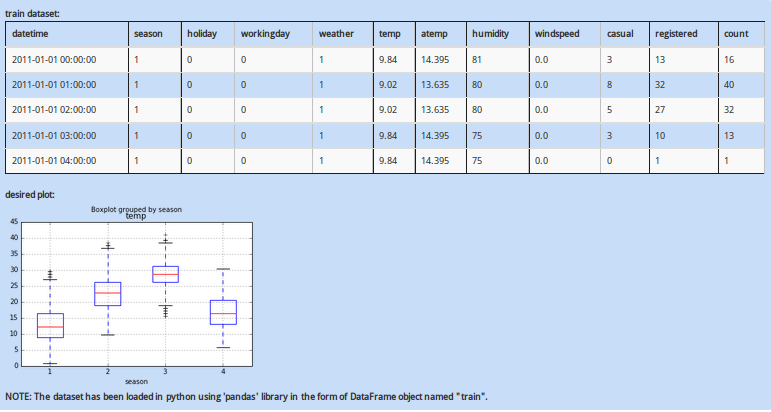One of the hypothesis for the data above is that seasonal variation of temperature could affect our target variable “Count”.

To visualize this, we could use a boxplot of based on the required columns.

Which of the following code will create the boxplot as shown above?

`A - train.boxplot(column='season', by='temp')`
`B - train.boxplot(ax='temp', by='season')`
`C - train.boxplot(ax='temp', column='season')`

`D - train.boxplot(column='temp', by='season')`

Solution: D

Q 42)One way to visualize frequency of variables in a column is to plot a histogram. Histograms give a rough sense of the density of the underlying data.

How will you plot a histogram of column ‘temp’ with bin size as ’50’?

`A - train.hist(column='temp')`
`B - train.hist(column='temp', bin_size=50)`
`C - train.hist(column='temp', bins=50)`

D – None of these

Solution: C

Q 43)
There is a method to check randomness in time series problem. If you plot an autocorrelation plot, you will see that uf time series is non-random then one or more of the autocorrelations will be significantly non-zero.
Here,  how would you code an autocorrelation plot for “temp” column?
`A - pd.tools.plotting.autocorr(train.temp)`
`B - pd.tools.plot.autocorr(train.temp)`
`C - pd.tools.plotting.autocorrelation_plot(train.temp)`

D – None of these

Solution: C

Q 44)

The plot is given for day wise distribution of the total no of rentals at every hour of the day first being Monday and last Sunday (transverse through rows).,

Fill the blanks with correct instructions to create the daywise_rental plot :

`>>> fig=plt.figure()`
`>>> for i in range(0,7):```` >>>     fig.add_subplot(3,3,____) >>>     t1=train[train['______']==i] >>>     t1.________(['hour'])['count'].sum().plot(kind='bar')```

In options day is the new variable created by extracting day of the week from datetime variable.

`A - i+1, day, groupby`
`B - i, day, groupby`
`C - i, Count, groupby`

`D - i, day, value_counts`

Solution: A

We want to output the total no of girls and boys data for the year 1880. Fill the blanks in the code for the following output :

```Gender
F     942
M    1058```

`>>> train.________(['Year','Gender']).size()._____`

`A - groupby, idx`
`B - groupby, loc`
`C - groupby, iloc`
`D - value_counts, iloc`

Solution: B

## End Notes

I hope you enjoyed this taking the skilltest and going through the detailed solution. I tried my best to make the solutions as comprehensive as possible but if you have any questions / doubts please drop in your comments below. And I would like to hear your feedback about the skilltest what you liked and what you think can be improved. Feel free to share them in comments below.

You can also read this article on our Mobile APP•zhongkailv says:

不错不错

•Faizan Shaikh says:

Thanks!

•Daniel Tschick Tomaz says:

I took the test on sunday and it was very cool!

Unfortunately, the test got stuck in question 25. As I clicked to go to the next question it kept going back to question 25 forever.

I think I learned a bit more about Python and Analytics by taking the test. Keep on with this events!

Greetings

•Faizan Shaikh says:

Its great that you liked the skill test! The reason you got stuck is because the time allotted for the skilltest had ended, so the server shutdown. We are trying to make the experience more smooth in the future. Stay tuned!

•Hari Galla says:

Amazing questions I learnt a lot from this skill test. I like so much thanks for posting Many more to come

•Faizan Shaikh says:

Thanks Hari! Stay tuned for more

•Python_Guy says:

where can I find this ‘Train’ dataset to practice?

•Faizan Shaikh says:

Hi Python_Guy, the table included in the question is the “train” dataset. Please read the note included in questions.